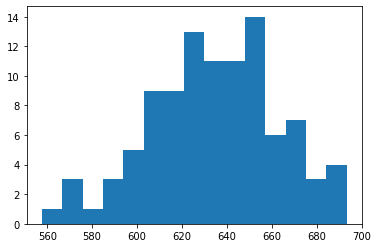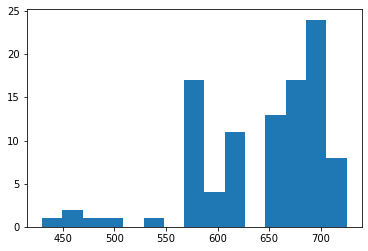# Model-Agnostic Uncertainty Estimates through Bootstrapping

A key element of a trustworthy model is that it can give an estimate of its confidence in a given prediction. We've already talked about one way to do this for linear models, and today we'll talk about a technique for getting uncertainty estimates for any model.

Let's continue using the fish dataset from last time:

```import os
import pandas as pd

```

We build a `ColumnTransformer` for convenience:

```from sklearn.compose import ColumnTransformer
from sklearn.preprocessing import StandardScaler, OneHotEncoder

ct = ColumnTransformer(
[
("scale", StandardScaler(), ["Length1", "Length2", "Length3", "Height", "Width"]),
("ohe", OneHotEncoder(), ["Species"]),
]
)
```

Next we construct a pipeline which uses the `ColumnTransformer` from above as well as `scikit-learn`'s implementation of bagging. Specifically, our `BaggingRegressor` will consist of 100 ElasticNet models, each one trained on a random 25% of the dataset (with replacement).

```from sklearn.ensemble import BaggingRegressor
import sklearn.linear_model as lm

pipe = make_pipeline(
ct, BaggingRegressor(lm.ElasticNetCV(), n_estimators=100, max_samples=0.25, random_state=42, n_jobs=-1,)
)

pipe.fit(fish, fish["Weight"])
```

Finally, we can snag those 100 models and make a prediction for a new fish:

```from sklearn.ensemble import BaggingRegressor
import sklearn.linear_model as lm

pipe = make_pipeline(
ct, BaggingRegressor(lm.ElasticNetCV(), n_estimators=100, max_samples=0.25, random_state=42, n_jobs=-1,)
)

pipe.fit(fish, fish["Weight"])

new_fish = pd.DataFrame(
[
{
"Species": "Bream",
"Weight": -1,
"Length1": 31.3,
"Length2": 34,
"Length3": 39.5,
"Height": 15.1285,
"Width": 5.5695,
}
]
)

predictions = [e.predict(new_fish) for e in estimators]

plt.hist(predictions, bins=15)
plt.savefig("twm1_hist.png", bbox_inches="tight")
```

Which gives us a nifty histogram of expected weight:The cool thing about this approach, though, is that we can swap in any model within the `BaggingRegressor`, and the rest of the code is unaffected. For instance, here's the distribution of predictions when using decision trees:Interesting idea, right? There's still a few more approaches I want to highlight in coming posts, but after that I'll be comparing them all to see which uncertainty estimation technique is best.